## Try to prove a stronger result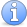Incomplete This article is incomplete. This article is still missing several examples and discussion

### Quick description

When we first set about trying to prove a result in mathematics, it can often be unclear which direction to take. If we try to prove a stronger result there is less room to manoeuvre and the proof can be easier to find.

### Example 1: Every Subgroup of the Integers under Addition is Cyclic

Suppose we are trying to prove that every subgroup of the integers under addition is cyclic. Appealing to the definition of cyclic groups, we can see that what we are trying to prove is the assertion that for every group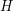that is a subgroup of the integers under addition, there exists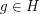such that for every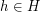,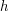is some power of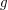. (Since we are talking about a group under addition, we interpret 'power of' as 'multiple of g'.)

How can we find a stronger result that will be easier to prove. In fact, how can we find a stronger result that can be proven at all - after all, many stronger results, such as the result that 'all groups are cyclic' are false. Well, a useful technique for finding stronger results which are always true is to assume the result you are trying to prove and see what you can derive from it. If we are going to be able to prove this result at all, it must be true, so any result we can derive from it must be true as well.

So, bearing this in mind, let us consider a subgroupof the integers under addition for which we have somesuch that for all,is a multiple of. What can we say about? Let's assume thatis positive. (We are justified in doing this: if a negative number generates, its inverse - which is positive- must also generate;cannot generate.) Now, if we consider only those elements ofwhich are positive, we can see that, since they are all positive multiples of, they must all be greater than or equal to. Somust be the smallest positive number in. This means that we can reformulate the result we are trying to prove as the following lemma, which is a stronger result:

Lemma 1 (The Strong Lemma) Every element of every subgroupof the integers under addition is a multiple of the smallest positive number in.

Proof of Lemma 1. This turns out to be quite easy to prove. The proof is by contradiction: letbe the smallest positive number inand assume on the contrary that there is some element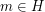with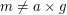for all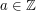. Now we can write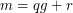for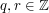,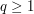,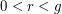. Clearly every positive multiple ofis contained within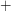is a binary operation on, so we can addto itself any number of times to create a new element of– so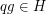. Therefore, the inverse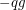is in. So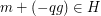. Since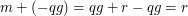,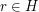. Butis positive and less than, and we had supposedto be the smallest positive element in. So we have derived a contradiction. So every element inis a multiple of.

The result that every subgroup of the integers under addition is cyclic clearly follows from Lemma 1.

### General discussion

In this case, we derived the stronger result from the result we were trying to prove. This special case of proving a stronger result is discussed more fully in the article Prove a consequence first.